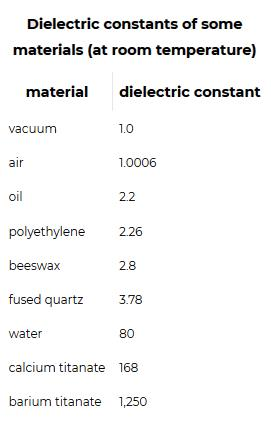Courses

# Dielectrics, Polarization, and Electric Dipole Moment Physics Notes | EduRev

## Physics : Dielectrics, Polarization, and Electric Dipole Moment Physics Notes | EduRev

The document Dielectrics, Polarization, and Electric Dipole Moment Physics Notes | EduRev is a part of the Physics Course Basic Physics for IIT JAM.
All you need of Physics at this link: Physics

The amount of charge stored in a capacitor is the product of the voltage and the capacity. What limits the amount of charge that can be stored on a capacitor? The voltage can be increased, but electric breakdown will occur if the electric field inside the capacitor becomes too large. The capacity can be increased by expanding the electrode areas and by reducing the gap between the electrodes. In general, capacitors that can withstand high voltages have a relatively small capacity. If only low voltages are needed, however, compact capacitors with rather large capacities can be manufactured. One method for increasing capacity is to insert between the conductors an insulating material that reduces the voltage because of its effect on the electric field. Such materials are called dielectrics (substances with no free charges). When the molecules of a dielectric are placed in the electric field, their negatively charged electrons separate slightly from their positively charged cores. With this separation, referred to as polarization, the molecules acquire an electric dipole moment. A cluster of charges with an electric dipole moment is often called an electric dipole.
Is there an electric force between a charged object and uncharged matter, such as a piece of wood? Surprisingly, the answer is yes, and the force is attractive. The reason is that under the influence of the electric field of a charged object, the negatively charged electrons and positively charged nuclei within the atoms and molecules are subjected to forces in opposite directions. As a result, the negative and positive charges separate slightly. Such atoms and molecules are said to be polarized and to have an electric dipole moment. The molecules in the wood acquire an electric dipole moment in the direction of the external electric field. The polarized molecules are attracted toward the charged object because the field increases in the direction of the charged object.
The electric dipole moment p of two charges +q and −q separated by a distance l is a vector of magnitude p = ql with a direction from the negative to the positive charge. An electric dipole in an external electric field is subjected to a torque τ = pE sin θ, where θ is the angle between p and E. The torque tends to align the dipole moment p in the direction of E. The potential energy of the dipole is given by Ue = −pE cos θ, or in vector notation Ue = −p · E. In a nonuniform electric field, the potential energy of an electric dipole also varies with position, and the dipole can be subjected to a force. The force on the dipole is in the direction of increasing field when p is aligned with E, since the potential energy Ue decreases in that direction.

The polarization of a medium P gives the electric dipole moment per unit volume of the material; it is expressed in units of coulombs per metre squared. When a dielectric is placed in an electric field, it acquires a polarization that depends on the field. The electric susceptibility χe relates the polarization to the electric field as P = χeE. In general, χe varies slightly depending on the strength of the electric field, but for some materials, called linear dielectrics, it is a constant. The dielectric constant κ of a substance is related to its susceptibility as κ = 1 + χe0; it is a dimensionless quantity. Table 1 lists the dielectric constants of a few substances.The presence of a dielectric affects many electric quantities. A dielectric reduces by a factor K the value of the electric field and consequently also the value of the electric potential from a charge within the medium. As seen in Table 1, a dielectric can have a large effect. The insertion of a dielectric between the electrodes of a capacitor with a given charge reduces the potential difference between the electrodes and thus increases the capacitance of the capacitor by the factor K. For a parallel-plate capacitor filled with a dielectric, the capacity becomes C = Κε0A/d. A third and important effect of a dielectric is to reduce the speed of electromagnetic waves in a medium by the factor Square root of√K .
Capacitors come in a wide variety of shapes and sizes. Not all have parallel plates; some are cylinders, for example. If two plates, each one square centimetre in area, are separated by a dielectric with Κ = 2 of one millimetre thickness, the capacity is 1.76 × 10−12 F, about two picofarads. Charged to 20 volts, this capacitor would store about 40 picocoulombs of charge; the electric energy stored would be 400 picojoules. Even small-sized capacitors can store enormous amounts of charge. Modern techniques and dielectric materials permit the manufacture of capacitors that occupy less than one cubic centimetre and yet store 1010 times more charge and electric energy than in the above example.

Offer running on EduRev: Apply code STAYHOME200 to get INR 200 off on our premium plan EduRev Infinity!

## Basic Physics for IIT JAM

109 videos|157 docs|94 tests

,

,

,

,

,

,

,

,

,

,

,

,

,

,

,

,

,

,

,

,

,

,

,

,

,

,

,

;